# Cable

Cable consists of 8 strands, each strand consists of 12 wires with diameter d = 0.5 mm. Calculate the cross-section of the cable.

Correct result:

S =  18.8 mm2

#### Solution: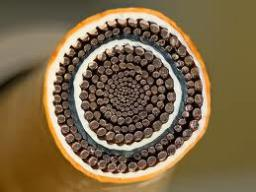We would be pleased if you find an error in the word problem, spelling mistakes, or inaccuracies and send it to us. Thank you!#### You need to know the following knowledge to solve this word math problem:

We encourage you to watch this tutorial video on this math problem:

## Next similar math problems:

• IronIron ore contains 57% iron. How much ore is needed to produce 20 tons of iron?
• Clock handsThe second hand has a length of 1.5 cm. How long does the endpoint of this hand travel in one day?
• CircleWhat is the radius of the circle whose perimeter is 6 cm?
• Circle - easy 2The circle has a radius 6 cm. Calculate:
• Two circlesTwo circles with a radius 4 cm and 3 cm have a center distance 0.5cm. How many common points have these circles?
• Acreage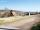Plot has a diamond shape, its side is 25.6 m long and the distance of the opposite sides is 22.2 meters. Calculate its acreage.
• SidewalkThe city park is a circular bed of flowers with a diameter of 8 meters, around it the whole length is 1 meter wide sidewalk . What is the sidewalk area?
• Circle area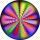Calculate the circle area with a radius of 1.2 m.
• AnnulusThe radius of the larger circle is 8cm, the radius of smaller is 5cm. Calculate the contents of the annulus.
• Calculate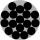Calculate the area of a circle whose radius is 5 dm.
• Area of ditchHow great content area will have a section of trapezoidal ditch with a width of 1.6 meters above and below 0.57 meters? The depth of the ditch is 2.08 meters.
• Diameter to areaFind the area of a circle whose diameter is 26cm.
• CD disc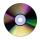A compact disc has a diameter of 11.8 cm. What is the surface area of the disc in square centimeters?
• Circle - simpleCalculate the area of a circle in dm2, if its circumference is 31.4 cm.
• 22/7 circleCalculate approximately area of a circle with radius 20 cm. When calculating π use 22/7.
• LawnsBefore a sports hall are two equally large rectangular lawns, each measuring 40 m and 12 m. Maintenance of 10m² lawn cost 45 CZK yearly. On each lawn is circular flowerbed with a diameter 8 meters. How much money is needed each year to take on lawn care?
• Pipe cross sectionThe pipe has an outside diameter 1100 mm and the pipe wall is 100 mm thick. Calculate the cross section of this pipe.